import pandas as pd
import numpy as np
import matplotlib.pyplot as plt
import seaborn as sns

plt.rcParams['figure.figsize'] = (8, 8)


## Feature extraction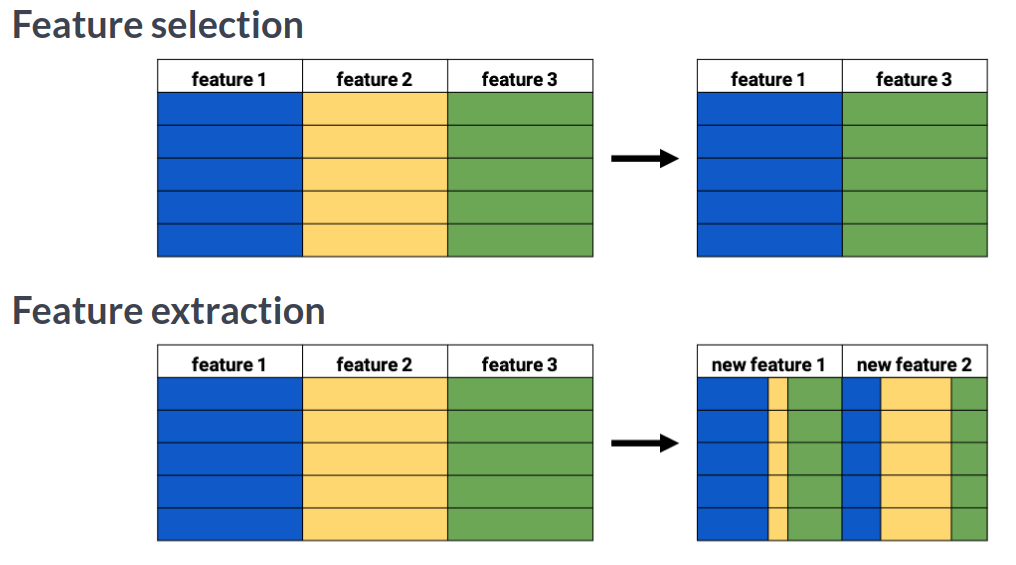### Manual feature extraction I

You want to compare prices for specific products between stores. The features in the pre-loaded dataset sales_df are: storeID, product, quantity and revenue. The quantity and revenue features tell you how many items of a particular product were sold in a store and what the total revenue was. For the purpose of your analysis it's more interesting to know the average price per product.

sales_df = pd.read_csv('./dataset/grocery_sales.csv')

storeID product quantity revenue
0 A Apples 1811 9300.6
1 A Bananas 1003 3375.2
2 A Oranges 1604 8528.5
3 B Apples 1785 9181.0
4 B Bananas 944 3680.2
sales_df['price'] = sales_df['revenue'] / sales_df['quantity']

# Drop the quantity and revenue features
reduced_df = sales_df.drop(['revenue', 'quantity'], axis=1)


storeID product price
0 A Apples 5.135616
1 A Bananas 3.365105
2 A Oranges 5.317020
3 B Apples 5.143417
4 B Bananas 3.898517

### Manual feature extraction II

You're working on a variant of the ANSUR dataset, height_df, where a person's height was measured 3 times. Add a feature with the mean height to the dataset, then drop the 3 original features.

height_df = pd.read_csv('./dataset/height_df.csv')

weight_kg height_1 height_2 height_3
0 81.5 1.78 1.80 1.80
1 72.6 1.70 1.70 1.69
2 92.9 1.74 1.75 1.73
3 79.4 1.66 1.68 1.67
4 94.6 1.91 1.93 1.90
height_df['height'] = height_df[['height_1', 'height_2', 'height_3']].mean(axis=1)

# Drop the 3 original height features
reduced_df = height_df.drop(['height_1', 'height_2', 'height_3'], axis=1)


weight_kg height
0 81.5 1.793333
1 72.6 1.696667
2 92.9 1.740000
3 79.4 1.670000
4 94.6 1.913333

## Principal component analysis

• PCA concept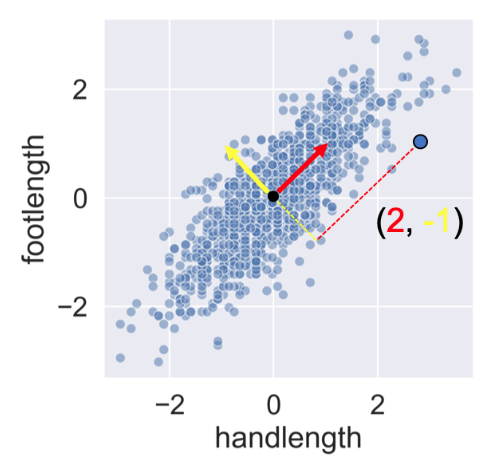### Calculating Principal Components

You'll visually inspect a 4 feature sample of the ANSUR dataset before and after PCA using Seaborn's pairplot(). This will allow you to inspect the pairwise correlations between the features.

ansur_df = pd.read_csv('./dataset/ansur_sample.csv')

sns.pairplot(ansur_df);from sklearn.preprocessing import StandardScaler
from sklearn.decomposition import PCA

# Create the scaler and standardize the data
scaler = StandardScaler()
ansur_std = scaler.fit_transform(ansur_df)

# Create the PCA instance and fit and transform the data with pca
pca = PCA()
pc = pca.fit_transform(ansur_std)

# This changes the numpy array output back to a dataframe
pc_df = pd.DataFrame(pc, columns=['PC 1', 'PC 2', 'PC 3', 'PC 4'])

# Create a pairplot of the pricipal component dataframe
sns.pairplot(pc_df);Notice how, in contrast to the input features, none of the principal components are correlated to one another.

### PCA on a larger dataset

You'll now apply PCA on a somewhat larger ANSUR datasample with 13 dimensions. The fitted model will be used in the next exercise. Since we are not using the principal components themselves there is no need to transform the data, instead, it is sufficient to fit pca to the data.

df = pd.read_csv('./dataset/ANSUR_II_MALE.csv')
ansur_df = df[['stature_m', 'buttockheight', 'waistdepth', 'span',
'waistcircumference', 'shouldercircumference', 'footlength',
'handlength', 'functionalleglength', 'chestheight',
'chestcircumference', 'cervicaleheight', 'sittingheight']]

scaler = StandardScaler()
ansur_std = scaler.fit_transform(ansur_df)

# Apply PCA
pca = PCA()
pca.fit(ansur_std)

PCA(copy=True, iterated_power='auto', n_components=None, random_state=None,
svd_solver='auto', tol=0.0, whiten=False)

You've fitted PCA on our 13 feature datasample. Now let's see how the components explain the variance.

### PCA explained variance

You'll be inspecting the variance explained by the different principal components of the pca instance you created in the previous exercise.

print(pca.explained_variance_ratio_)

[0.57832831 0.2230137  0.06404218 0.04252456 0.0278581  0.01761021
0.01681037 0.01014147 0.00706488 0.00607973 0.00344643 0.00228095
0.00079911]

print(pca.explained_variance_ratio_.cumsum())

[0.57832831 0.801342   0.86538419 0.90790875 0.93576684 0.95337706
0.97018743 0.9803289  0.98739378 0.99347351 0.99691994 0.99920089
1.        ]


Based on the data, we can use 4 principal components if we don't want to lose more than 10% of explained variance during dimensionality reduction.

## PCA applications

### Understanding the components

You'll apply PCA to the numeric features of the Pokemon dataset, poke_df, using a pipeline to combine the feature scaling and PCA in one go. You'll then interpret the meanings of the first two components.

df = pd.read_csv('./dataset/pokemon.csv')

# Name Type 1 Type 2 Total HP Attack Defense Sp. Atk Sp. Def Speed Generation Legendary
0 1 Bulbasaur Grass Poison 318 45 49 49 65 65 45 1 False
1 2 Ivysaur Grass Poison 405 60 62 63 80 80 60 1 False
2 3 Venusaur Grass Poison 525 80 82 83 100 100 80 1 False
3 3 VenusaurMega Venusaur Grass Poison 625 80 100 123 122 120 80 1 False
4 4 Charmander Fire NaN 309 39 52 43 60 50 65 1 False
poke_df = df[['HP', 'Attack', 'Defense', 'Sp. Atk', 'Sp. Def', 'Speed']]

HP Attack Defense Sp. Atk Sp. Def Speed
0 45 49 49 65 65 45
1 60 62 63 80 80 60
2 80 82 83 100 100 80
3 80 100 123 122 120 80
4 39 52 43 60 50 65
from sklearn.pipeline import Pipeline

# Build the pipeline
pipe = Pipeline([
('scaler', StandardScaler()),
('reducer', PCA(n_components=2))
])

# Fit it to the dataset and extract the component vectors
pipe.fit(poke_df)
vectors = pipe.steps.components_.round(2)

# Print feature effects
print('PC 1 effects = ' + str(dict(zip(poke_df.columns, vectors))))
print('PC 2 effects = ' + str(dict(zip(poke_df.columns, vectors))))

PC 1 effects = {'HP': 0.39, 'Attack': 0.44, 'Defense': 0.36, 'Sp. Atk': 0.46, 'Sp. Def': 0.45, 'Speed': 0.34}
PC 2 effects = {'HP': 0.08, 'Attack': -0.01, 'Defense': 0.63, 'Sp. Atk': -0.31, 'Sp. Def': 0.24, 'Speed': -0.67}


In PC1, All features have a similar positive effect. PC 1 can be interpreted as a measure of overall quality (high stats). In contrast, PC2's defense has a strong positive effect on the second component and speed a strong negative one. This component quantifies an agility vs. armor & protection trade-off.

### PCA for feature exploration

You'll use the PCA pipeline you've built in the previous exercise to visually explore how some categorical features relate to the variance in poke_df. These categorical features (Type & Legendary) can be found in a separate dataframe poke_cat_df.

poke_cat_df = df[['Type 1', 'Legendary']]

pipe = Pipeline([
('scaler', StandardScaler()),
('reducer', PCA(n_components=2))
])

# Fit the pipeline to poke_df and transform the data
pc = pipe.fit_transform(poke_df)

print(pc)

[[-1.5563747  -0.02148212]
[-0.36286656 -0.05026854]
[ 1.28015158 -0.06272022]
...
[ 2.45821626 -0.51588158]
[ 3.5303971  -0.95106516]
[ 2.23378629  0.53762985]]

poke_cat_df.loc[:, 'PC 1'] = pc[:, 0]
poke_cat_df.loc[:, 'PC 2'] = pc[:, 1]


/home/chanseok/anaconda3/lib/python3.7/site-packages/pandas/core/indexing.py:844: SettingWithCopyWarning:
A value is trying to be set on a copy of a slice from a DataFrame.
Try using .loc[row_indexer,col_indexer] = value instead

See the caveats in the documentation: https://pandas.pydata.org/pandas-docs/stable/user_guide/indexing.html#returning-a-view-versus-a-copy
self.obj[key] = _infer_fill_value(value)
/home/chanseok/anaconda3/lib/python3.7/site-packages/pandas/core/indexing.py:965: SettingWithCopyWarning:
A value is trying to be set on a copy of a slice from a DataFrame.
Try using .loc[row_indexer,col_indexer] = value instead

See the caveats in the documentation: https://pandas.pydata.org/pandas-docs/stable/user_guide/indexing.html#returning-a-view-versus-a-copy
self.obj[item] = s

Type 1 Legendary PC 1 PC 2
0 Grass False -1.556375 -0.021482
1 Grass False -0.362867 -0.050269
2 Grass False 1.280152 -0.062720
3 Grass False 2.620916 0.704263
4 Fire False -1.758284 -0.706179
sns.scatterplot(data=poke_cat_df, x='PC 1', y='PC 2', hue='Type 1');sns.scatterplot(data=poke_cat_df, x='PC 1', y='PC 2', hue='Legendary');Looks like the different types are scattered all over the place while the legendary Pokemon always score high for PC 1 meaning they have high stats overall. Their spread along the PC 2 axis tells us they aren't consistently fast and vulnerable or slow and armored.

### PCA in a model pipeline

We just saw that legendary Pokemon tend to have higher stats overall. Let's see if we can add a classifier to our pipeline that detects legendary versus non-legendary Pokemon based on the principal components.

from sklearn.ensemble import RandomForestClassifier
from sklearn.model_selection import train_test_split

X = poke_df
y = df['Legendary']

X_train, X_test, y_train, y_test = train_test_split(X, y, test_size=0.3, random_state=0)

pipe = Pipeline([
('scaler', StandardScaler()),
('reducer', PCA(n_components=2)),
('classifier', RandomForestClassifier(random_state=0))
])

# Fit the pipeline to the training data
pipe.fit(X_train, y_train)

# Prints the explained variance ratio
print(pipe.steps.explained_variance_ratio_)

# Score the acuracy on the test set
accuracy = pipe.score(X_test, y_test)

# Prints the model accuracy
print('{0:.1%} test set accuracy'.format(accuracy))

[0.45673596 0.18599109]
92.1% test set accuracy


Repeat the process with 3 extracted components.

pipe = Pipeline([
('scaler', StandardScaler()),
('reducer', PCA(n_components=3)),
('classifier', RandomForestClassifier(random_state=0))])

# Fit the pipeline to the training data
pipe.fit(X_train, y_train)

# Score the accuracy on the test set
accuracy = pipe.score(X_test, y_test)

# Prints the explained variance ratio and accuracy
print(pipe.steps.explained_variance_ratio_)
print('{0:.1%} test set accuracy'.format(accuracy))

[0.45673596 0.18599109 0.12852181]
93.8% test set accuracy

pipe = Pipeline([
('scaler', StandardScaler()),
('reducer', PCA(n_components=4)),
('classifier', RandomForestClassifier(random_state=0))])

# Fit the pipeline to the training data
pipe.fit(X_train, y_train)

# Score the accuracy on the test set
accuracy = pipe.score(X_test, y_test)

# Prints the explained variance ratio and accuracy
print(pipe.steps.explained_variance_ratio_)
print('{0:.1%} test set accuracy'.format(accuracy))

[0.45673596 0.18599109 0.12852181 0.11442161]
95.0% test set accuracy


## Principal Component Selection

• PCA operations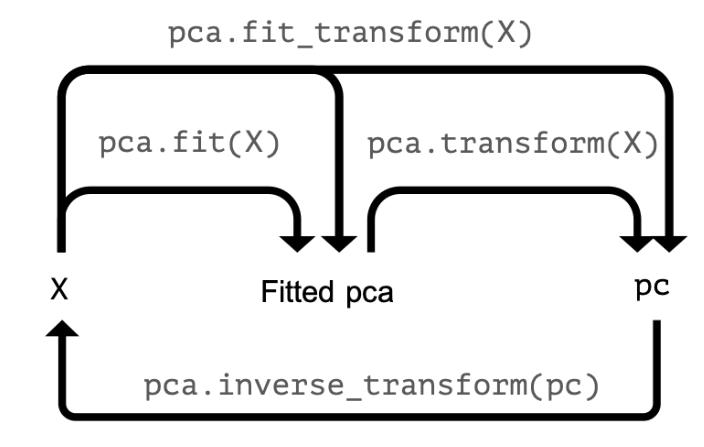### Selecting the proportion of variance to keep

You'll let PCA determine the number of components to calculate based on an explained variance threshold that you decide.

ansur_df = pd.read_csv('./dataset/ANSUR_II_FEMALE.csv')

Branch Component Gender abdominalextensiondepthsitting acromialheight acromionradialelength anklecircumference axillaheight balloffootcircumference balloffootlength ... waistdepth waistfrontlengthsitting waistheightomphalion wristcircumference wristheight weight_kg stature_m BMI BMI_class Height_class
0 Combat Support Regular Army Female 231 1282 301 204 1180 222 177 ... 217 345 942 152 756 65.7 1.560 26.997041 Overweight Normal
1 Combat Service Support Regular Army Female 194 1379 320 207 1292 225 178 ... 168 329 1032 155 815 53.4 1.665 19.262506 Normal Normal
2 Combat Service Support Regular Army Female 183 1369 329 233 1271 237 196 ... 159 367 1035 162 799 66.3 1.711 22.647148 Normal Tall
3 Combat Service Support Regular Army Female 261 1356 306 214 1250 240 188 ... 235 371 999 173 818 78.2 1.660 28.378575 Overweight Normal
4 Combat Arms Regular Army Female 309 1303 308 214 1210 217 182 ... 300 380 911 152 762 88.6 1.572 35.853259 Overweight Normal

5 rows × 99 columns

ansur_df.drop(['Gender', 'Branch', 'Component', 'BMI_class', 'Height_class'],
axis=1, inplace=True)
ansur_df.shape

(1986, 94)
pipe = Pipeline([
('scaler', StandardScaler()),
('reducer', PCA(n_components=0.8))
])

# Fit the pipe to the data
pipe.fit(ansur_df)

print('{} components selected'.format(len(pipe.steps.components_)))

11 components selected

pipe = Pipeline([
('scaler', StandardScaler()),
('reducer', PCA(n_components=0.9))
])

# Fit the pipe to the data
pipe.fit(ansur_df)

print('{} components selected'.format(len(pipe.steps.components_)))

23 components selected


From the result, we need more than 12 components to go from 80% to 90% explained variance.

### Choosing the number of components

You'll now make a more informed decision on the number of principal components to reduce your data to using the "elbow in the plot" technique.

pipe = Pipeline([
('scaler', StandardScaler()),
('reducer', PCA(n_components=10))
])

# Fit the pipe to the data
pipe.fit(ansur_df)

# Plot the explained variance ratio
plt.plot(pipe.steps.explained_variance_ratio_);
plt.xlabel('Principal component index');
plt.ylabel('Explained variance ratio');
plt.title('Elbow plot of Explained variance ratio');
plt.grid(True);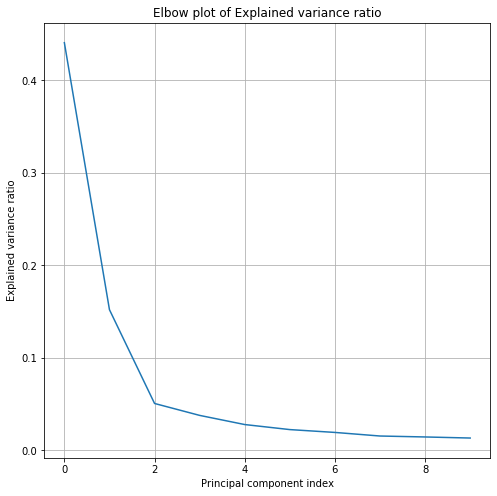### PCA for image compression

You'll reduce the size of 16 images with hand written digits (MNIST dataset) using PCA.

The samples are 28 by 28 pixel gray scale images that have been flattened to arrays with 784 elements each (28 x 28 = 784) and added to the 2D numpy array X_test. Each of the 784 pixels has a value between 0 and 255 and can be regarded as a feature.

A pipeline with a scaler and PCA model to select 78 components has been pre-loaded for you as pipe. This pipeline has already been fitted to the entire MNIST dataset except for the 16 samples in X_test.

def plot_digits(data):
fig, axes = plt.subplots(4, 4, figsize=(6, 6),
subplot_kw={'xticks':[], 'yticks':[]},
gridspec_kw=dict(hspace=0.05, wspace=0.05))
for i, ax in enumerate(axes.flat):
ax.imshow(data[i].reshape(28, 28),
cmap='binary',
clim=(0, 300))

from sklearn.datasets import fetch_openml

X, y = X, y = fetch_openml('mnist_784', version=1, return_X_y=True)
X_train, X_test, y_train, y_test = train_test_split(X, y, test_size=0.3)
X_sample = X_test[:1600:100]

pipe = Pipeline([
('scaler', StandardScaler()),
('reducer', PCA(n_components=78))
])

pipe.fit(X_train)

Pipeline(memory=None,
steps=[('scaler',
StandardScaler(copy=True, with_mean=True, with_std=True)),
('reducer',
PCA(copy=True, iterated_power='auto', n_components=78,
random_state=None, svd_solver='auto', tol=0.0,
whiten=False))],
verbose=False)
plot_digits(X_sample)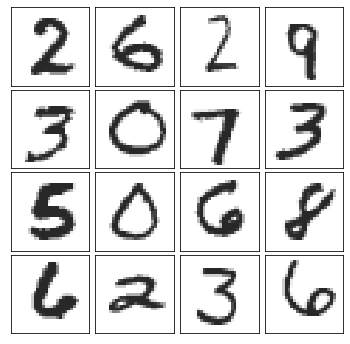pc = pipe.transform(X_sample)

# Prints the number of features per dataset
print("X_test has {} features".format(X_sample.shape))
print("pc has {} features".format(pc.shape))

X_test has 784 features
pc has 78 features

X_rebuilt = pipe.inverse_transform(pc)

# Prints the number of features
print("X_rebuilt has {} features".format(X_rebuilt.shape))

X_rebuilt has 784 features

plot_digits(X_rebuilt)You've reduced the size of the data 10 fold but were able to reconstruct images with reasonable quality.